# java实现简单二叉树

24 篇文章 4 订阅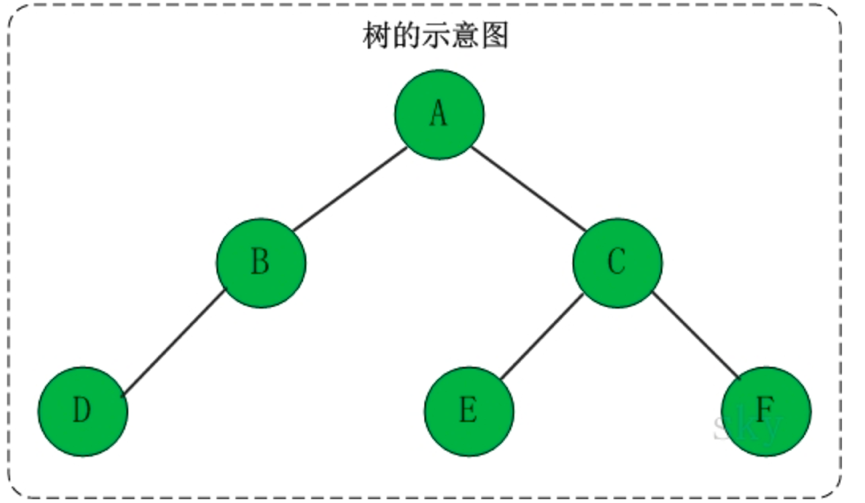（1）每个结点有零个或多个子结点

（2）没有父节点的结点称为根节点

（3）每一个非根结点有且只有一个父节点

（4）除了根结点外，每个子结点可以分为多个不相交的子树。

1、二叉树的定义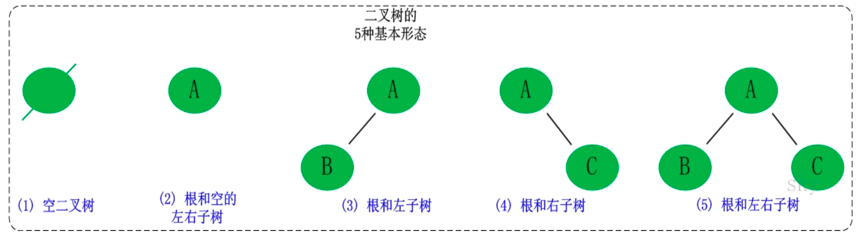2、二叉树的性质

3、性质4的证明

1、满二叉树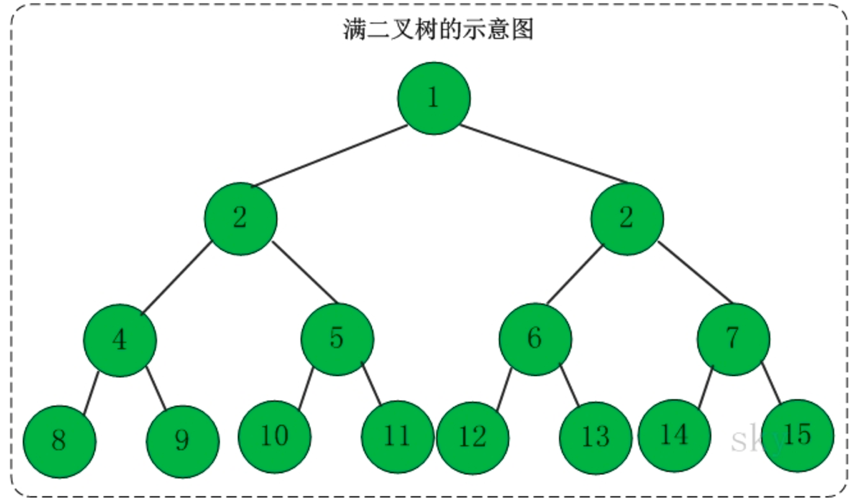2、完全二叉树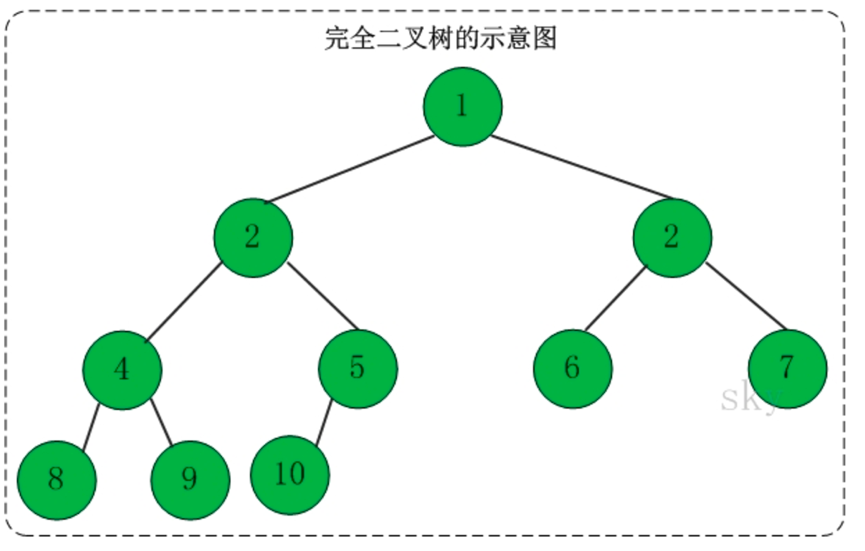3、二叉查找树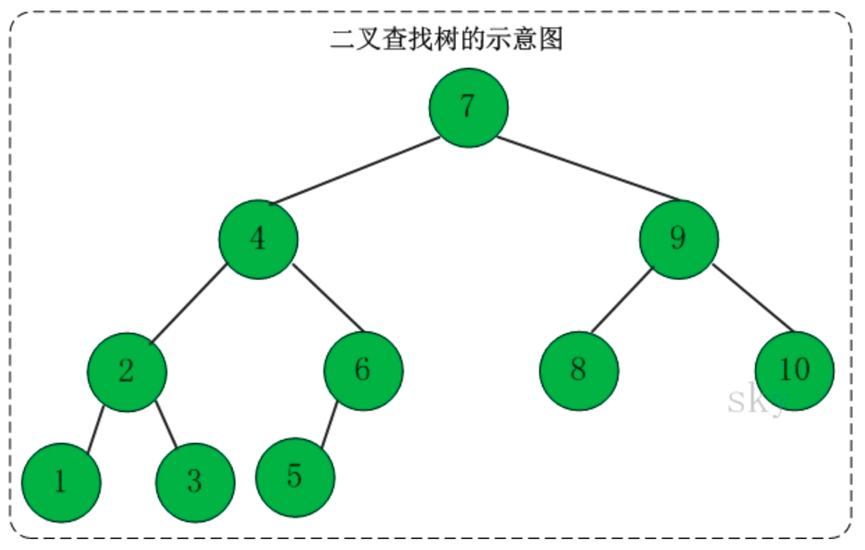（1）若任意结点的左子树不空，则左子树上所有结点的值均小于它的根结点的值。

（2）任意结点的右子树不空，则右子树上所有结点的值均大于它的根结点的值。

（3）任意结点的左、右子树也分别为二叉查找树。

（4）没有键值相等的结点。

import java.util.ArrayList;
import java.util.List;

public class bintree {
public bintree left;
public bintree right;
public bintree root;
//    数据域
private Object data;
//    存节点
public List<bintree> datas;

public bintree(bintree left, bintree right, Object data){
this.left=left;
this.right=right;
this.data=data;
}
//    将初始的左右孩子值为空
public bintree(Object data){
this(null,null,data);
}

public bintree() {

}

public void creat(Object[] objs){
datas=new ArrayList<bintree>();
//        将一个数组的值依次转换为Node节点
for(Object o:objs){
}
//        第一个数为根节点
root=datas.get(0);
//        建立二叉树
for (int i = 0; i <objs.length/2; i++) {
//            左孩子
datas.get(i).left=datas.get(i*2+1);
//            右孩子
if(i*2+2<datas.size()){//避免偶数的时候 下标越界
datas.get(i).right=datas.get(i*2+2);
}

}

}
//先序遍历
public void preorder(bintree root){
if(root!=null){
System.out.println(root.data);
preorder(root.left);
preorder(root.right);
}

}
//中序遍历
public void inorder(bintree root){
if(root!=null){
inorder(root.left);
System.out.println(root.data);
inorder(root.right);
}

}
//    后序遍历
public void afterorder(bintree root){
if(root!=null){
System.out.println(root.data);
afterorder(root.left);
afterorder(root.right);
}

}

public static void main(String[] args) {
bintree bintree=new bintree();
Object []a={2,4,5,7,1,6,12,32,51,22};
bintree.creat(a);
bintree.preorder(bintree.root);
}
}

import java.util.Scanner;

public class btree {
private btree left,right;
private char data;
public btree creat(String des){
Scanner scanner=new Scanner(System.in);
System.out.println("des:"+des);
String str=scanner.next();
if(str.charAt(0)<'a')return null;
btree root=new btree();
root.data=str.charAt(0);
root.left=creat(str.charAt(0)+"左子树");
root.right=creat(str.charAt(0)+"右子树");
return root;
}
public void midprint(btree btree){
//        中序遍历
if(btree!=null){
midprint(btree.left);
System.out.print(btree.data+"  ");
midprint(btree.right);
}
}
public void firprint(btree btree){
//        先序遍历
if(btree!=null){
System.out.print(btree.data+" ");
firprint(btree.left);
firprint(btree.right);
}
}
public void lastprint(btree btree){
//        后序遍历
if(btree!=null){
lastprint(btree.left);
lastprint(btree.right);
System.out.print(btree.data+"  ");
}
}

public static void main(String[] args) {
btree tree = new btree();
btree newtree=tree.creat("根节点");
tree.firprint(newtree);
System.out.println();
tree.midprint(newtree);
System.out.println();
tree.lastprint(newtree);
}
}

des:根节点
a
des:a左子树
e
des:e左子树
c
des:c左子树
1
des:c右子树
1
des:e右子树
b
des:b左子树

1
des:b右子树
1
des:a右子树
d
des:d左子树
f
des:f左子树
1
des:f右子树
1
des:d右子树
1
a e c b d f        先序
c  e  b  a  f  d   中序
c  b  e  f  d  a   后序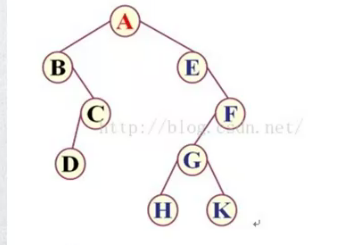前序遍历：ABCDEFGHK

中序遍历：BDCAEHGKF

后序遍历：DCBHKGFEA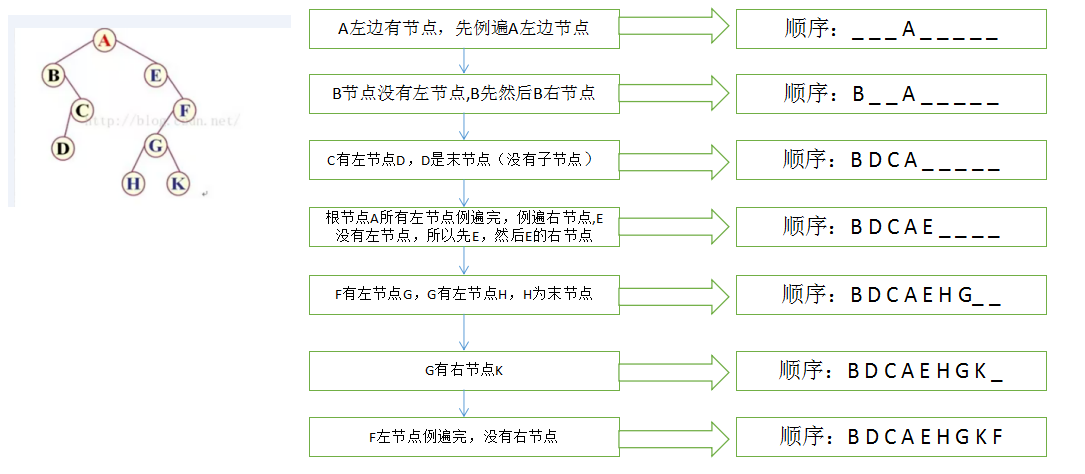07-1403-271万+
10-02342
09-02
10-097787
08-023万+
10-31254
07-0927
02-102260
06-102万+
10-204581
07-18
03-1613
08-19639
08-261056
01-04348
01-15287点击重新获取扫码支付余额充值